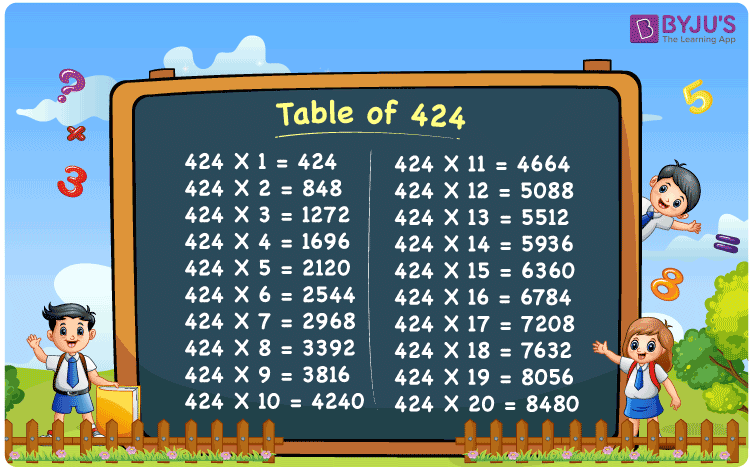Checkout JEE MAINS 2022 Question Paper Analysis : Checkout JEE MAINS 2022 Question Paper Analysis :

# Table of 424

The Multiplication Table for 424 is nothing more than the repetitive addition of the number 424. Repetitive addition is adding the same number multiple times. For example, adding 4 times 424, which is 424 + 424 + 424 + 424 is the same as 424 x 4 and that equals 1696. But adding the same number multiple times, say 8 times, can result in mistakes. Instead, the below-given chart on Table 424 can be referred to. Any multiplication table can be computed using the Multiplication Calculator.

## Table of 424 Chart## What is the 424 Times Table?

In the table of 424, we are repeatedly adding 424 multiple times. And the table below gives a repetitive addition 10 times for the number 424.

 424 × 1 = 424 424 424 × 2 = 848 424 + 424 = 848 424 × 3 = 1272 424 + 424 + 424 = 1272 424 × 4 = 1696 424 + 424 + 424 + 424 = 1696 424 × 5 = 2120 424 + 424 + 424 + 424 + 424 = 2120 424 × 6 = 2544 424 + 424 + 424 + 424 + 424 + 424 = 2544 424 × 7 = 2968 424 + 424 + 424 + 424 + 424 + 424 + 424 = 2968 424 × 8 = 3392 424 + 424 + 424 + 424 + 424 + 424 + 424 + 424 = 3392 424 × 9 = 3816 424 + 424 + 424 + 424 + 424 + 424 + 424 + 424 + 424 = 3816 424 × 10 = 4240 424 + 424 + 424 + 424 + 424 + 424 + 424 + 424 + 424 + 424 = 4240

## Multiplication Table of 424

Given below is the multiplication table of 424 being done 20 times. The below table helps you with multiplication problems that require the tables of 424.

 424 × 1 = 424 424 × 2 = 848 424 × 3 = 1272 424 × 4 = 1696 424 × 5 = 2120 424 × 6 = 2544 424 × 7 = 2968 424 × 8 = 3392 424 × 9 = 3816 424 × 10 = 4240 424 × 11 = 4664 424 × 12 = 5088 424 × 13 = 5512 424 × 14 = 5936 424 × 15 = 6360 424 × 16 = 6784 424 × 17 = 7208 424 × 18 = 7632 424 × 19 = 8056 424 × 20 = 8480

## Solved Example on Table of 424

Example:

Solve for y:

424 y – 300 = 6060

Solution: y = 15

424 y – 300 = 6060

424 y = 6060 + 300

424 y = 6360

y = 6360 / 424

y = 15.

## Frequently Asked Questions on Table of 424

### Question 1: What is 16 times 424?

Answer: 16 times 424 is 6784.

### Question 2: What are the factors of 424?

Answer: The factors of 424 are 1, 2, 4, 8, 53, 106, 212 and 424.

### Question 3: What are the prime factors of 424?

Answer: 2 and 53 are the prime factors of 424.